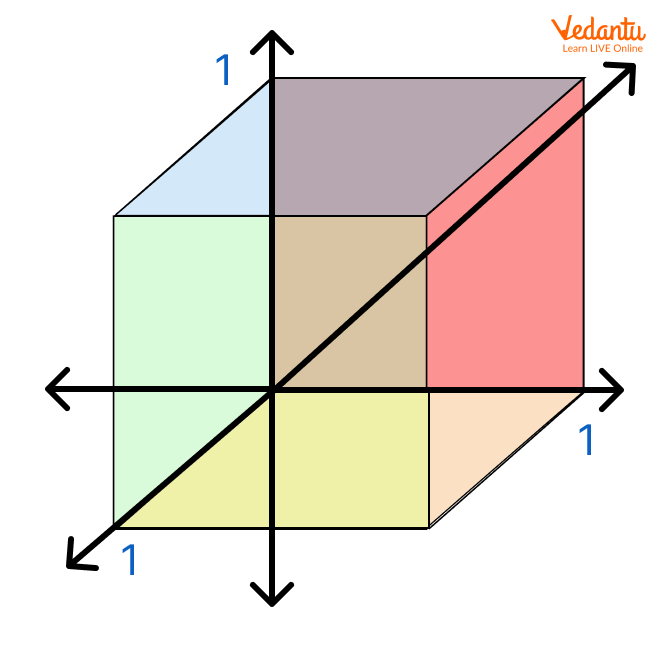Courses
Courses for Kids
Free study material
Offline Centres
More

# Unit Cube and Cube: Learning Maths with Fun for KidsLast updated date: 25th Nov 2023
Total views: 150k
Views today: 1.50k## Cube and Unit Cube - What Are They?

Have you ever played the ludo? Yes! That is great. Then surely you have used dice. Isn't it? Can you tell the shape of a dice? Or, you might try to fix the Rubik’s Cube. What is the shape of a Rubik’s cube? The shape of both the objects is a ‘cube’. A cube is an object that has all edges equal in size. Can you count the number of edges a cube has? Yes, you are right, it is 12.

But the question is what is a unit cube? Let's read the complete article and get the answers to such questions.

## What Is the Unit Cube?

Like any other cube, a unit cube is a three-dimensional object. It also has 12 edges. And, the major point to be focussed on is that the length of all edges is equal to 1 unit. A unit cube is also called a ‘Cube of Side 1’. It has six faces which are unit squares.Unit Cube

## The Volume of a Unit Cube

We know that the volume of a cube is calculated as:

Volume = side x side x side = (side)3

To evaluate the volume of a unit cube, we know that the side of a cube is 1 unit. Therefore,

Volume of unit cube = 1 x 1 x 1 = 13= 1

∴ The volume of unit cube = 1 unit3 or 1 cubic unit.

So, the volume of a cube is 1 cubic unit.

Now, let us calculate the surface area of a unit cube.

## Surface Area of Unit Cube

A unit cube has 6 faces, and the dimension of each side is 1 unit. All the faces are square in shape.

So, the area of each face = Side x Side

= 1 unit x 1 unit

= 1 square unit

A unit cube has 6 faces of 1 square unit each.

Therefore, the total surface area of unit square = 6 x area of one face of a unit square

= 6 x 1 square unit

= 6 square units

Hence, the surface of the unit cube is 6 square units.

## Do You Know?

• A unit cube is used to calculate the density of any object. (How much mass an object has of one unit cube is known as the density of any object.)

• It is also used to calculate the volume of other cubic or cuboidal objects. (We can calculate it by filling or building the object using the unit cube.)

## Numerical Type Questions on Cubes

Question 1. What is the volume of an object that is made up of combining the six unit cubes?

Solution: The new shape is made by combining the six unit cubes.

We know that the volume of a unit cube is 1 cubic unit.

Therefore, the volume of the new object = 6 x 1 cubic unit

= 6 cubic unit

Question 2: What is the density of an object of mass 500 kg which occupies a space of a unit cube?

Solution: We know that the density of an object is equal to the mass of an object occupied by one unit cube.

So, density = $\frac{Mass}{Volume}$

Density = $\frac{500kg}{1 cubic unit}$

Density = 500 kg unit-3

## A Tip for Parents

We request all parents to introduce their children to geometrical shapes. Show them daily-life examples of different shapes and their applications. Introducing different shapes not only helps them to learn the concept deeply but also opens further dimensions to increase their quantitative and numerical ability.

## Conclusion

In this article, we have learnt about the cube and unit cube. We have also derived the value of the volume and surface area of a unit cube.

## FAQs on Unit Cube and Cube: Learning Maths with Fun for Kids

1. What is a unit cube? How many sides does it have?

A unit cube is a three-dimensional object. It has six faces and 12 sides. The length of all sides is 1 unit and the area of each face is one square unit.  If we look at a unit cube from any view, then it looks like a square.

2. What is a cube? What are its related formulae?

A cube is a three-dimensional object. It has 12 edges, 12 vertices, and 6 faces. Some examples of a cube are Rubik’s cube, dice, etc. All sides of a cube are equal in size, and all faces have an equal area.

Some of the formulae related to cube are the following:

1. Volume of a cube = side x side x side = (side)3

2. Total surface area of cube = 6 x (side)2